Courses

# Energy Equation And Its Applications - 2

## 9 Questions MCQ Test Mock Test Series for Civil Engineering (CE) GATE 2020 | Energy Equation And Its Applications - 2

Description
This mock test of Energy Equation And Its Applications - 2 for Civil Engineering (CE) helps you for every Civil Engineering (CE) entrance exam. This contains 9 Multiple Choice Questions for Civil Engineering (CE) Energy Equation And Its Applications - 2 (mcq) to study with solutions a complete question bank. The solved questions answers in this Energy Equation And Its Applications - 2 quiz give you a good mix of easy questions and tough questions. Civil Engineering (CE) students definitely take this Energy Equation And Its Applications - 2 exercise for a better result in the exam. You can find other Energy Equation And Its Applications - 2 extra questions, long questions & short questions for Civil Engineering (CE) on EduRev as well by searching above.
QUESTION: 1

Solution:
QUESTION: 2

### If the coefficient of velocity of flow through a orifice is cv and coefficient of resistance is cr, then

Solution: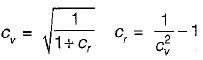QUESTION: 3

### If Cv, Cc, Dd and Cr are the hydraulic coefficients of an orifice, then

Solution:
QUESTION: 4

The momentum correction factor for triangular velocity distribution for flow between parallel plates as shown in the figure is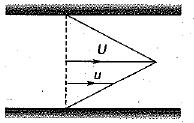Solution:

Triangular velocity distribution is achieved for Couette flow.
Average velocity,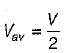Momentum correction factor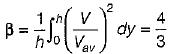Energy correction factor,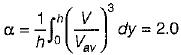QUESTION: 5

If the velocity is zero over half of the cross- sectionai area and is uniform over the remaining half, then the momentum correction factor is

Solution:

Momentum correction factor,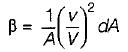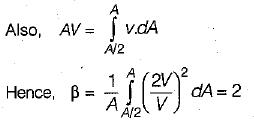QUESTION: 6

Match List-I with List-I and select the correct answer using the codes given below the lists:
List-I
A. Moment of momentum equation
B. Bernoulli’s equation
C. Euler’s equation
D. Hagen-Poiseuille equation

List-II
1. Equation to find energy loss in a pipeline
2. Equation of motion for one-dimensional steady flow of ideal and incompressible fluid
3. Equation based on conservation of momentum principle applicable to circulatory flows
4. Three-dimensional equation ofmotion based on Principle of conservation of momentum for ideal and incompressible fluid flow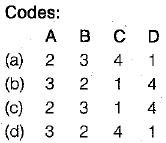Solution:

Bernoulli's equation is derived from equation of motion for one-dimensional steady flow of ideal and incompressible fluid. \

QUESTION: 7

If velocity is zero over 1/3rd of a cross-section and is uniform over remaining 2/3rd of the cross-section, then the correction factor for kinetic energy is

Solution: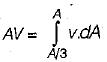∴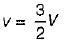∴ Kinetic energy correction factor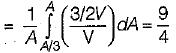QUESTION: 8

A jet of water issue from a 5cm diameter nozzle, held vertically upwards, at a velocity of 20 m/sec. If air resistance consumes 10% of the initial energy of the jet, then it would reach a height, above the nozzle, of

Solution:

Using Bernoulli’s equation,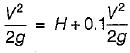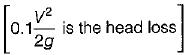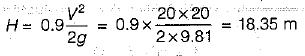QUESTION: 9

The kinetic energy correction factor is

Solution: